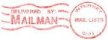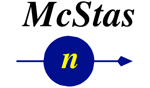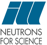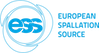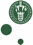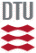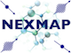McStas: Source_gen Component

# The `Source_gen` Component

Circular/squared neutron source with flat or Maxwellian energy/wavelength spectrum

## Identification

• Author: Emmanuel Farhi, Kim Lefmann
• Origin: ILL/Risoe
• Date: Aug 27, 2001
• Version:(Unknown)
• Modification history:
• EF, Aug 27, 2001 ; can use Energy/wavelength and I1
• EF, Sep 18, 2001 ; corrected illumination bug. Add options
• EF, Oct 30, 2001 ; minor changes. mccompcurname replaced

## Description

```This routine is a neutron source (rectangular or circular), which aims at
a square target centered at the beam (in order to improve MC-acceptance
rate). The angular divergence is then given by the dimensions of the
target. However, it may be directly set using the 'focus-aw' and 'focus_ah'
parameters.

The neutron energy/wavelength is distributed uniformly in wavelength between
Emin=E0-dE and Emax=E0+dE or Lmin=lambda0-dlambda and Lmax=lambda0+dlambda.
The I1 may be either arbitrary (I1=0), or specified in neutrons per steradian
per square cm per Angstrom per s. A Maxwellian spectra may be selected if you
give the source temperatures (up to 3).

Finally, a file with the flux as a
function of the wavelength [lambda(AA) flux(n/s/cm^2/st/AA)] may be used
with the 'flux_file' parameter. Format is 2 columns free text.

Additional distributions for the horizontal and vertical phase spaces
distributions (position-divergence) may be specified with the
'xdiv_file' and 'ydiv_file' parameters. Format is free text, requiring
a comment line '# xylimits: pos_min pos_max div_min div_max' to set
the axis of the distribution matrix. All these files may be generated using
standard monitors (better in McStas/PGPLOT format), e.g.:
Monitor_nD(options="auto lambda per cm2")
Monitor_nD(options="x hdiv, all auto")
Monitor_nD(options="y vdiv, all auto")

The source shape is defined by its radius, or can alternatively be squared
if you specify non-zero yheight and xwidth parameters.
The beam is divergence uniform,.
The source may have a thickness, which will broaden the default zero time
distribution.

Usage example:
Source_gen(yheight=0.1,xwidth=0.1,Emin=1,Emax=3,I1=1e13,verbose=1,focus_xw=0.01,focus_yh=0.01)
EXTEND
%{
t = rand0max(1e-3); // set time from 0 to 1 ms for TOF instruments.
%}

Some neutron facility parameters:
PSI cold source     T1=296.2,I1=8.5E11, T2=40.68,I2=5.2E11
ILL VCS cold source T1=216.8,I1=1.24e+13,T2=33.9,I2=1.02e+13
(H1, 58 MW)  T3=16.7 ,I3=3.0423e+12
ILL HCS cold source T1=413.5,I1=10.22e12,T2=145.8,I2=3.44e13
(H5, 58 MW)  T3=40.1 ,I3=2.78e13
ILL Thermal tube    T1=683.7,I1=0.5874e+13,T2=257.7,I2=2.5099e+13
(H12, 58 MW) T3=16.7 ,I3=1.0343e+12
ILL Hot source      T1=1695, I1=1.74e13,T2=708,  I2=3.9e12 (58MW)
HZB cold source     T1=43.7 ,I1=1.4e12, T2=137.2,I2=2.08e12,radius=.155 (10MW)
HZB bi-spectral     T1=43.7, I1=1.4e12, T2=137.2,I2=2.08e12,T3=293.0,I3=1.77e12
HZB thermal tube    T1=293.0,I1=2.64e12 (10MW)
FRM2 cold,20MW      T1=35.0, I1=9.38e12,T2=547.5,I2=2.23e12,T3=195.4,I3=1.26e13
FRM2 thermal,20MW   T1=285.6,I1=3.06e13,T2=300.0,I2=1.68e12,T3=429.9,I3=6.77e12
LLB cold,14MW       T1=220,  I1=2.09e12,T2=60,   I2=3.83e12,T3=20,   I3=1.04e12
TRIGA thermal 1MW   T1=300,  I1=3.5e11 (scale by thermal power in MW)

%VALIDATION
Feb 2005: output cross-checked for 3 Maxwellians against VITESS source
I(lambda), I(hor_div), I(vert_div) identical in shape and absolute values
Validated by: K. Lieutenant

```

## Input parameters

Parameters in boldface are required; the others are optional.
Name Unit Description Default
flux_file str Name of a two columns [lambda flux] text file that contains the wavelength distribution of the flux in either [1/(s*cm**2*st)] or [1/(s*cm**2*st*AA)] (see flux_file_perAA flag) Comments (#) and further columns are ignored. Format is compatible with McStas/PGPLOT wavelength monitor files. When specified, temperature and intensity values are ignored. "NULL"
xdiv_file str Name of the x-horiz. divergence distribution file, given as a free format text matrix, preceeded with a line '# xylimits: xmin xmax xdiv_min xdiv_max' "NULL"
ydiv_file str Name of the y-vert. divergence distribution file, given as a free format text matrix, preceeded with a line '# xylimits: ymin ymax ydiv_min ydiv_max' "NULL"
radius m Radius of circle in (x,y,0) plane where neutrons are generated. You may also use 'yheight' and 'xwidth' for a square source 0.0
dist m Distance to target along z axis. 0
focus_xw m Width of target. 0.045
focus_yh m Height of target. 0.12
focus_aw deg maximal (uniform) horz. width divergence 0
focus_ah deg maximal (uniform) vert. height divergence 0
E0 meV Mean energy of neutrons. 0
dE meV Energy spread of neutrons, half width. 0
lambda0 AA Mean wavelength of neutrons. 0
dlambda AA Wavelength spread of neutrons,half width 0
I1 1/(cm**2*sr) Source flux per solid angle, area and Angstrom if I1=0, the source emits 1 in 4*PI whole space. 1
yheight m Source y-height, then does not use radius parameter 0.1
xwidth m Source x-width, then does not use radius parameter 0.1
verbose 0/1 display info about the source. -1 unactivate source. 0
T1 K Temperature of the Maxwellian source, 0=none 0
flux_file_perAA 1 When true (1), indicates that flux file data is already per Aangstroem. If false, file data is per wavelength bin. 0
flux_file_log 1 When true, will transform the flux table in log scale to improve the sampling. 0
Lmin AA Minimum wavelength of neutrons 0
Lmax AA Maximum wavelength of neutrons 0
Emin meV Minimum energy of neutrons 0
Emax meV Maximum energy of neutrons 0
T2 K Second Maxwellian source Temperature, 0=none 0
I2 1/(cm**2*sr) Second Maxwellian Source flux 0
T3 K Third Maxwellian source Temperature, 0=none 0
I3 1/(cm**2*sr) Third Maxwellian Source flux 0
zdepth m Source z-zdepth, not anymore flat 0
target_index 1 relative index of component to focus at, e.g. next is +1 this is used to compute 'dist' automatically. +1

## Output parameters

Name Unit Description Default
p_in
lambda1
lambda2
lambda3
pTable
pTable_x
pTable_y
pTable_xmin
pTable_xmax
pTable_xsum
pTable_ymin
pTable_ymax
pTable_ysum
pTable_dxmin
pTable_dxmax
pTable_dymin
pTable_dymax

• Source code for `Source_gen.comp`.